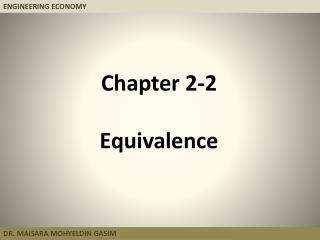DownloadDownload PresentationChapter 2-2 Equivalence

# Chapter 2-2 Equivalence

Download Presentation## Chapter 2-2 Equivalence

- - - - - - - - - - - - - - - - - - - - - - - - - - - E N D - - - - - - - - - - - - - - - - - - - - - - - - - - -
##### Presentation Transcript

1. Chapter 2-2 Equivalence

2. Today’s Objectives: • By the end of this lecture students will be able to • 1- understand Equivalence in engineering economy.

3. Equivalence Two cash flow streams are said to be equivalent at k% interest if and only if their present worths are equal at k% interest.

4. Equivalence Example What uniform series over periods [1,8] is equivalent at 15% to the following cash flow profile?

5. Equivalence Example What uniform series over periods [1,8] is equivalent at 15% to the following cash flow profile? Solution: [100(F|P 15%,7)+200(F|P 15%,5)+100(F|P 15%,4) +300(F|P 15%,3)](A|F 15%,8) = \$94.86 Answer: \$94.86

6. Example 2.28 What single sum at t=6 is equivalent at 10% to the following cash flow profile?

7. Example 2.28 What single sum at t=6 is equivalent at 10% to the following cash flow profile? Solution: [-400+100(P|A 10%,3)](F|P 10%,5) + 100(P|A 10%,3)(F|P 10%,1) = \$29.85 Answer: \$29.85

8. Example 2.28(Alternative Solution) What single sum of money at t=6 is equivalent to the following cash flow profile if i = 10%? Solution: F = [\$100(F|A 10%,7)-\$400(F|P 10%,7)-\$100(F|P 10%,3)](P|F 10%,2) F = [\$100(9.48717)-\$400(1.94872)-\$100(1.33100)](0.82645) F = \$29.86 Answer: \$29.86

9. Example 2.29 What uniform series over [1,5] is equivalent to the following cash flow profile if i = 8%?

10. Example 2.29 What uniform series over [1,5] is equivalent to the following cash flow profile if i = 8%? Solution: The uniform series equivalent over [2,6] is A = \$500 - \$100(A|G 8%,5) or \$500 - \$100(1.84647) = \$315.35 The uniform series equivalent over [1,5] is A = \$315.35(P|F 8%,1) or \$315.35(0.92593) = \$291.99 Answer: \$291.99

11. Example 2.30 FW(LHS) = \$200(F|A 15%,4) + \$100(F|A 15%,3) + \$100 FW(RHS) = [\$200 + X(A|G 15%,4)](F|A 15%,4) Equating the two and eliminating the common term of \$200(F|A 15%,4), \$100(3.47250) + \$100 = X(1.32626)(4.99338) Solving for X give a value of \$67.53. Determine the value of X that makes the two CFDs equivalent.

12. Example 2.31 For what interest rate are the two cash flow diagrams equivalent?

13. Example 2.31(Continued) For what interest rate are the two cash flow diagrams equivalent? -\$4000(A|P i%,5) + \$1500 = -\$7000(A|P i%,5) + \$1500 + \$500(A|G i%,5) i≈ 13.8641% (by interpolation)